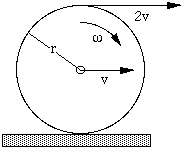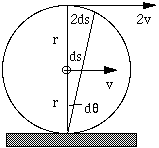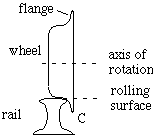# Rolling: the physics of wheels

This page supports the multimedia tutorials Weight and Contact Forces and Rotation.

 What happens when a wheel rolls? What part of a train is going twice as fast as the train? What part of a train is going backwards? The animation at right traces the path (purple curve) of a point on the rim of a rolling wheel. This curve is called a cycloid.A wheel turns clockwise about its axis. We measure its angular velocity ω in radians per second. So the number of complete turns per second is ω/2π. If it rotates through angle dθ in time dt, then ω = dθ/dt Let a point on the rim of the wheel travel a distance ds in time dt, so v  = ds/dt. From the definition of angle, ds = rdθ, we get v = rdθ/dt,   or   v = rω The diagram shows that all points on the rim of the wheel are travelling at speed v with respect to the axle, although their directions are different. The top of the wheel is travelling to the right, the bottom to the left, and so on.
Ā

Now let's suppose that the wheel is rolling on the ground--let's imagine it as a bicycle wheel--and we insist that it is rolling, not skidding. The view we have presented above left is the view of the bike rider, who is travelling to the right in this case. With respect to the rider, the position of the axle is fixed, the top of the wheel is going forwards at v, and the bottom of the wheel is going backwards at v, as above.

Ā
 Now the difference between rolling and skidding is that, in rolling, there is no relative motion at the point of contact. To have a feeling for this (literally), do this experiment: first, roll a bottle or ball along your arm. Then drag it along your arm. In the first case, there is no (horizontal) relative motion between the contact of the bottle and your skin, whereas in the second case you feel the bottle slide over the skin.
Ā
 The point at the bottom of a rolling wheel is instantaneously stationary. The axle is moving forward at speed v so, from the geometry shown, the top of the wheel is moving forwards at 2v. We can also understand this in terms of relative velocities. To an observer standing on the road, the axle of the bicycle is travelling at v. The cyclist sees the bottom of the wheel travelling at -v (speed v backwards, see diagram above), so the observer sees this point as having velocity v-v = 0. The cyclist sees the top of the wheel 'overtaking' him at speed v, so the observer sees it moving at 2v.Looking more closely. Looking again at the animated graph, we can watch the variation in position and velocity of a point on the rim of the wheel. In the film clip, a piece of white tape on the rear tire labels such a point. Observe in particular the horizontal (and vertical) components of the displacement from one frame to the next. Let's be quantitative. For a point rotating clockwise in a circle with radius r at angular frequency ω, starting at the top of the circle, the coordinates are x = r sin ωt, y = r cos ωt (You may wish to check that this has its centre at (0,0) and that x 2+y2=r2.) To make it roll along the x axis, as in the animation, the axle should move to the right at speed v = rω, and should be r above the axis, so we put the centre of the rotation at (vt,r). So we raise all points by r, and we move them to the right at v = rω. The coordinates of the point initially at the top of the wheel (the point circled in the animation or the white tape on the wheel) are now x = rωt + r sin ωt, y = r + r cos ωt    or x = r(ωt  + sin ωt), y = r(1 + cos ωt) Let's now look at the horizontal component of the velocity of that point: dx/dt = r(ω + ω cos ωt) = rω(1 + cos ωt) The value of the cos function varies from -1 to +1. So dx/dt for this point, the horizontal component of the velocity, has a maximum value of 2r ω = 2v when it is at the top of the wheel--the top of the wheel is overtaking the axle with twice its speed. It has a minimum value of zero, when its value is instanteously zero as it touches the ground.
 Let's now answer the question: What part of a train goes backwards? The sketch at right shows a cross section of a railway wheel (not to scale). The flange around the rim of the wheel protrudes below the surface of the rail. Flanges inside the rails on both sides are what stops the train from leaving the tracks. Now the point of the wheel that touches the rail--the rolling surface--is instantaneously stationary, and the parts of the wheel above it, as with the bicycle wheel, are travelling forwards. But what about the part below the rail? The next animation shows this.The blue circle is the rolling surface of the wheel, the green circle is the edge of the flange. The small blue and green circles indentify points fixed on the wheel, and their motions are traced by the purple and red curves respectively. Point C in the diagram above corresponds to the lowest point of the red curve.This work is licensed under a Creative Commons License.